# GSEB Solutions Class 10 Maths Chapter 8 Introduction to Trigonometry Ex 8.1

Gujarat Board GSEB Solutions Class 10 Maths Chapter 8 Introduction to Trigonometry Ex 8.1 Textbook Questions and Answers.

## Gujarat Board Textbook Solutions Class 10 Maths Chapter 8 Introduction to Trigonometry Ex 8.1

Question 1.
In ΔABC, right angled at B, AB = 24 cm, BC = 7 cm, determine
(i) sin A, cos A
(ii) sin C, cos C
Solution:
We have
AB = 24 cm
BC = 7 cm

(i) In right ΔABC
⇒ AC2 = AB2 + BC2
⇒ AC2 = 242 + 72
⇒ AC2 = 576 + 49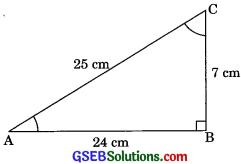⇒AC2 = 625
AC = 25 cm
san A = $$\frac {BC}{AC}$$
sin A = $$\frac {7}{25}$$
cos A = $$\frac {AB}{AC}$$ ⇒ cos A = $$\frac {24}{25}$$

(ii) sin C = $$\frac {AB}{AC}$$ ⇒ sin C = $$\frac {24}{25}$$
cos C = $$\frac {BC}{AC}$$ ⇒ cos C = $$\frac {7}{25}$$Question 2.
In Fig. find tan P – cot R.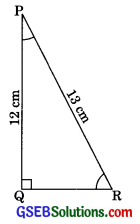Soution:
In ΔPQR
PR2 = PQ2 + QR2
⇒ 132 = 122 + QR2
⇒ 169 = 144 + QR2
⇒ QR2 = 169 – 144
⇒ QR2 = 25
⇒ QR = 5 cm
tan P = $$\frac {QR}{PR}$$ ⇒ tan P = $$\frac {5}{12}$$
⇒ cot R = $$\frac {QR}{PQ}$$ ⇒ cot R = $$\frac {5}{12}$$
∴ tan P – cot R = $$\frac {5}{12}$$ – $$\frac {5}{12}$$ = 0

Question 3.
If sin A = $$\frac {3}{4}$$ca1cu1ate cos A and tan A.
Solution:
We have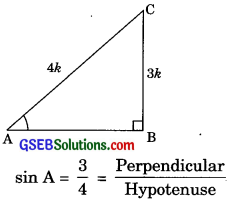BC = 3k
and AC = 4k
In right ΔABC
AC2 = AB2 + BC2
(4k)2 = AB2 + (3k)2
16k2 = AB2 + 9k2
AB2 – 16k2 – 9k2
AB2 – 7k2
AB = $$\sqrt{7}$$ k
∴ cos A = $$\frac{\mathrm{AB}}{\mathrm{AC}}$$ = $$\frac{\sqrt{7} k}{4 k}$$ = $$\frac{\sqrt{7}}{4}$$
And tan A = $$\frac{\mathrm{BC}}{\mathrm{AB}}$$ = $$\frac{3 k}{\sqrt{7} k}$$ = $$\frac{3}{\sqrt{7}}$$Question 4.
Given 15 cot A = 8 find sin A and sec A.
Solution:
Given 15 cot A = 8,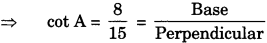AB = 8k
and BC = 15k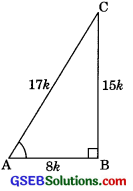In right ΔABC,
AC2 = AB2 + BC2
⇒ AC2 = (8k)2 +(15k)2
⇒ AC2 = 64k2 + 225k2
⇒ AC2 = 289k2
⇒ AC = 17k
∴ sin A = $$\frac {BC}{AC}$$ = $$\frac {15k}{17k}$$ = $$\frac {15}{17}$$
and sec A = $$\frac {AC}{AB}$$ = $$\frac {17k}{8k}$$ = $$\frac {17}{8}$$

Question 5.
Given sec θ = $$\frac {13}{12}$$calculate all other trigono metric ratios.
Solution:
We have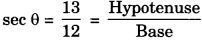AC = 13k and AB = 12k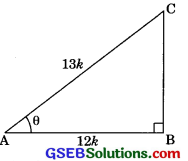In right ΔABC,
AC2 = AB2 + BC2
(13k)2 = (12k)2 + BC2
BC2 = 169k2 – 144k2
BC2 = 25k2
BC = 5k
sin θ = $$\frac {BC}{AC}$$ = $$\frac {5k}{13k}$$ = $$\frac {5}{13}$$
Cos θ = $$\frac {AB}{AC}$$ = $$\frac {12k}{13k}$$ = $$\frac {12}{13}$$
tan θ = $$\frac {BC}{AB}$$ = $$\frac {5k}{12k}$$ = $$\frac {5}{12}$$
cosec θ = $$\frac {AC}{BC}$$ = $$\frac {13k}{5k}$$ = $$\frac {13}{5}$$
cot θ = $$\frac {AB}{BC}$$ = $$\frac {12k}{5k}$$ = $$\frac {12}{5}$$Question 6.
1f ∠A and ∠B are acute angles such that cos A = cos B, then show that ∠A = ∠B.
Solution:
Let us consider two right triangles LMN and PQR.
cos A = cos B
$$\frac {PQ}{PR}$$ = $$\frac {LM}{LN}$$ = k Also = $$\frac {PQ}{LM}$$ = $$\frac {PR}{LN}$$ …….( 1)
(where k is a positive number)
PQ = kPR
LM = kLN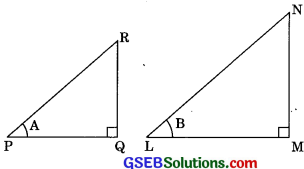Now, in ΔPQR
PR2 = PQ2 + QR2
⇒ PR2 = (kPR)2 + QR2
⇒ QR2 = PR2 – k2PR2
⇒ QR = $$\sqrt{\mathrm{PR}^{2}-k^{2} \mathrm{PR}^{2}}$$
⇒ QR = PR $$\sqrt{1-k^{2}}$$ ……….(2)
In right ΔLMN
LN2 = LM2 + MN2
⇒ LN2 = (kLN)2 + MN2
⇒ MN2 = LN2 – k2LN2
⇒ MN = $$\sqrt{\mathrm{L} \mathrm{N}^{2}-k^{2} \mathrm{L} \mathrm{N}^{2}}$$
⇒ MN = LN$$\sqrt{1-k^{2}}$$ ……….(3)
Dividing eqn. (2) by eqn. (3)
$$\frac {QR}{MN}$$ = $$\frac{\mathrm{PR} \sqrt{1-k^{2}}}{\mathrm{L} \mathrm{N} \sqrt{1-k^{2}}}$$ = $$\frac{Q R}{M N}=\frac{P R}{L N}$$ ………..(4)
From equation (1) and (4),
$$\frac{Q R}{M N}=\frac{P R}{L N}=\frac{P Q}{L M}$$
∴ Δ PQR – ΔLMN (by SSS similarity)
∴ ∠PQR = ∠LMN
(corresponding angle of two similar triangles)
∠A = ∠BQuestion 7.
If cot θ = $$\frac {7}{8}$$ evaluate:
(i) $$\frac{(1+\sin \theta)(1-\sin \theta)}{(1+\cos \theta)(1-\cos \theta)}$$
(ii) cot2 θ
Solution:
We have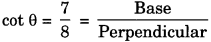AB = 7k and BC = 8k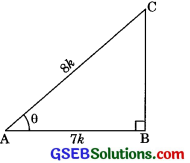In right ΔABC,
AC2 = AB2 + BC2
⇒ AC2 = (7k)2 + (8k)2 = 49k2 + 64k2
⇒ AC2 = 113k2
⇒ AC = $$\sqrt{113 k}$$
sin θ = $$\frac{\mathrm{BC}}{\mathrm{AC}}=\frac{8 k}{\sqrt{113 k}}=\frac{8}{\sqrt{113}}$$
cos θ = $$\frac{\mathrm{AB}}{\mathrm{AC}}=\frac{7 k}{\sqrt{133} k}=\frac{7}{\sqrt{133}}$$

(i) $$\frac{(1+\sin \theta)(1-\sin \theta)}{(1+\cos \theta)(1-\cos \theta)}$$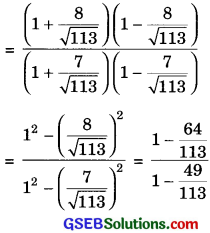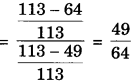(ii) cot2 θ = (cot θ)2
= ($$\frac {7}{8}$$)2 (cot θ = $$\frac {AB}{BC}$$ = $$\frac {7}{8}$$)
= $$\frac {49}{64}$$Question 8.
If 3 cot A =4, check whether
$$\frac{1-\tan ^{2} A}{1+\tan ^{2} A}$$ = cos2 A – sin2 A or not.
Solution:
We have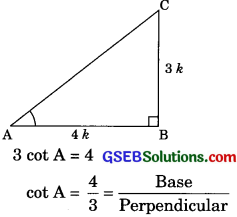⇒ AB = 4k
⇒ BC = 3k
In right ΔABC,
AC2 = AB2 + BC2
= (4k)2 + (3k)2
= 16k2 + 9k2
⇒ AC2 = 25k2
⇒ AC = 5k
tan A = $$\frac{\mathrm{BC}}{\mathrm{AB}}=\frac{3 k}{4 k}=\frac{3}{4}$$
sin A = $$\frac{\mathrm{BC}}{\mathrm{AC}}=\frac{3 k}{5 k}=\frac{3}{5}$$
cos A = $$\frac{A B}{A C}=\frac{4 k}{5 k}=\frac{4}{5}$$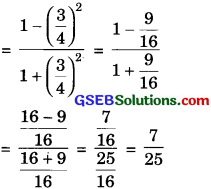RHS = cos2A – sin2A
= ($$\frac {4}{5}$$)2 – ($$\frac {4}{5}$$)2
= $$\frac{16}{25}-\frac{9}{25}=\frac{7}{25}$$
LHS = RHS

Question 9.
In AABC, right angled at B, if tan A = $$\frac{1}{\sqrt{3}}$$ ,find the value of
(j) sin A cos C + cos A sin C
(ii) cos A cos C – sin A sin C
Solution:
We have
tan A = $$\frac{1}{\sqrt{3}}$$
⇒ $$\frac{\mathrm{BC}}{\mathrm{AB}}=\frac{1}{\sqrt{3}}$$
⇒ $$\frac{\mathrm{BC}}{1}=\frac{\mathrm{AB}}{\sqrt{3}}$$ = k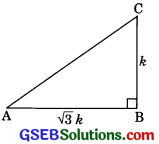∴ BC = k and AB = $$\sqrt{3} k$$

in right ΔABC
AC2 = AB2 + BC2
⇒ AC2 = ($$\sqrt{3} k$$)2 + k2
AC2 = 3k2 + k2
AC2 = 4k2
AC = 2k
Now sin A = $$\frac{\mathrm{BC}}{\mathrm{AC}}=\frac{k}{2 k}=\frac{1}{2}$$
Cos A = $$\frac{\mathrm{AB}}{\mathrm{AC}}=\frac{\sqrt{3} k}{2 k}=\frac{\sqrt{3}}{2}$$
sin C = $$\frac{\mathrm{AB}}{\mathrm{AC}}=\frac{\sqrt{3} k}{2 k}=\frac{\sqrt{3}}{2}$$
Cos C = $$\frac{\mathrm{BC}}{\mathrm{AC}}=\frac{k}{2 k}=\frac{1}{2}$$

(i) sin A cos C + cos A sin C
= $$\frac{1}{2} \times \frac{1}{2}+\frac{\sqrt{3}}{2} \times \frac{\sqrt{3}}{2}$$
= $$\frac{1}{4}+\frac{3}{4}=\frac{1+3}{4}=\frac{4}{4}=1$$

(ii) cos A cos C – sin A sin C
= $$\frac{\sqrt{3}}{2} \times \frac{1}{2}-\frac{1}{2} \times \frac{\sqrt{3}}{2}$$
= $$\frac{\sqrt{3}}{4}-\frac{\sqrt{3}}{4}$$ = 0Question 10.
In APQR, right-angled at Q, PR + QR = 25 cm and PQ = 5 cm. Determine the value of sin P, cos P and tan P.
Solution:
In right triangle PQR,
PR2 = PQ2 + QR2 ……..(1)
(By Pythagoras theorem)
PR + QR = 25
⇒ PR = 25 – QR ……….(2)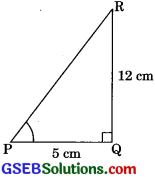From eqn. (1) and (2)
(25 – QR)2 = (5)2 + QR2
625 – 2 x 25 x QR + QR2
= 25 + QR2
= 625 – 5O x QR = 52
– 5O QR = 25 – 625
– 5O QR = – 600
QR = $$\frac{600}{50}$$ = 12
Putting in eqn. (2),
PR = 25 – QR = 25 – 12
PR = 13 cm
sin P = $$\frac{QR}{PR}$$ = $$\frac{12}{13}$$
cos P = $$\frac{P Q}{P R}=\frac{5}{13}$$
and tan P = $$\frac{Q R}{P Q}=\frac{12}{5}$$

Question 11.
(i) The value of tan A is always less than 1
(ii) sec A = $$\frac{12}{5}$$ for some value of A
(iii) cos A is the abbreviation used for the cosecant of angle A
(iv) cot A is the product of cot and A
(v) sin θ = $$\frac{4}{3}$$ for some angle.
Solution:
(i) False.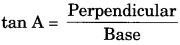the value of the perpendicular may be longer than the base.

(ii) True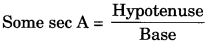Because Hypotenuse is always greater than the base.

(iii) False
Since cos A is the abbreviation used for the cosine of angle A.

(iv) False
Since cot is meaningless without angle A.

(v) False
Since value of sin O is always less than 1.